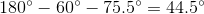# SAT II Math I : Finding Angles

## Example Questions

### Example Question #1 : Finding Angles

What angle do the minute and hour hands of a clock form at 4:45?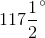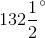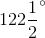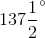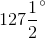Explanation:

There are twelve numbers on a clock; from one to the next, a hand rotates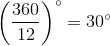. At 4:45, the minute hand is exactly on the "9" - that is, at the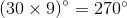. The hour hand is three-fourths of the way from the "4" to the "5" - that is, on the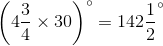position. Therefore, the difference is the angle they make: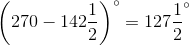### Example Question #2 : Finding Angles

What angle do the minute and hour hands of a clock form at 8:50?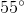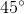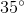Explanation:

There are twelve numbers on a clock; from one to the next, a hand rotates. At 8:50, the minute hand is exactly on the "10" - that is, on the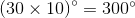position. The hour hand is five-sixth of the way from the "8" to the "9" - that is, on the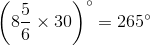position. Therefore, the difference is the angle they make: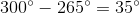.

### Example Question #3 : Finding Angles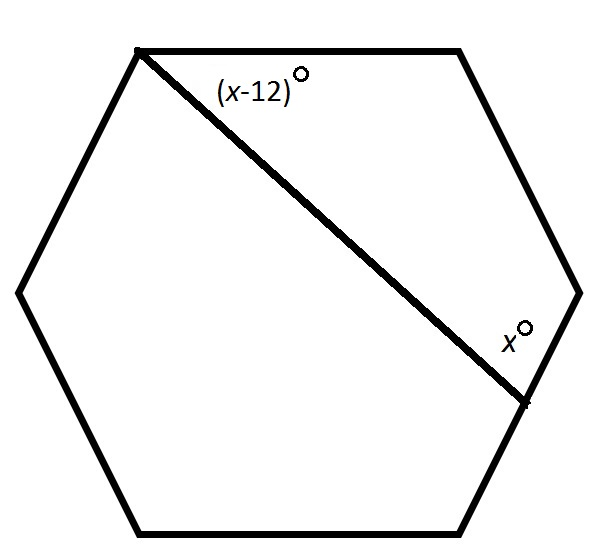Note: Figure NOT drawn to scale.

The above hexagon is regular. What is?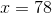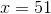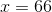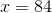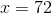Explanation:

Two of the angles of the quadrilateral formed are angles of a regular hexagon, so each measures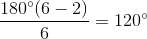.

The four angles of the quadrilateral are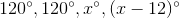. Their sum is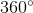, so we can set up, and solve forin, the equation: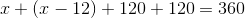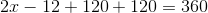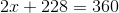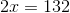### Example Question #4 : Finding Angles

Can a triangle have a set of angles that are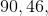anddegrees?

No

Yes

No

Explanation:

A triangle's angles must add up todegrees.

The angles given add up to 181,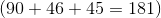.

That means that this cannot be an actual triangle.

### Example Question #5 : Finding Angles

If two angles of a triangle areand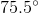, find the measurement of the third angle.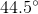Explanation:

Step 1: Recall the sum of the angles of a triangle...

The sum of the internal angles of a triangle is.

Step 2: To find the missing angle, subtract the given angles from...